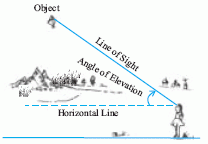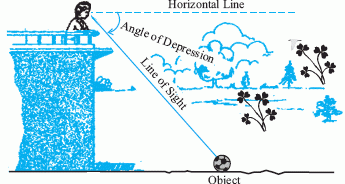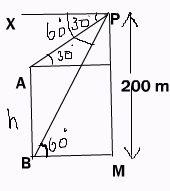Name: ___________________Date:___________________

 Email us to get an instant 20% discount on highly effective K-12 Math & English kwizNET Programs!

### High School Mathematics - 210.2 Heights and Distances

 Introduction One of the most important applications of trigonometry is the application of measurement of heights and distances which cannot be measured directly. It is also extensively used by astronomers in determining the distance of the heavenly bodies like the sun and moon and stars. Two angles are very often used in the practical applications of trigonometry . Line of sight The line of object is the line from our eyes to object. Angle of Elevation If the object is above the horizontal level of our eyes, we have to turn our head upwards to view the object. This is called the angle of elevation.Angle of Depression If the object is below the horizontal level of our eyes, we have to bend our head to view it. This is called the angle of elevation.Example 1: The angle of elevation of the top of a tower at a distance of 100 metres from its foot on a horizontal plane is found to be 60o. Find the height of the tower. Let CA be the tower equal to h metres in length and B point at a distance of 100 metres from its foot C. It is given that angle ABC = 60oFrom the right angle triangle ABC we have h/100 = tan 60 Solving the above we get h = 173.2 metres Answer: Height of the tower is 173.2 metres Example 2: From the top of a cliff, 200 metres high, the angle of depression of the top and bottom of a tower are observed to be observed to be 30o and 60o, find the height of the tower.Solution: Let AB represent the tower and P the top of cliff MP. If PX be the horizontal line through P, then angle XPA = 30o and angle XPB = 60o. Let the height of the tower be h metres. From A draw AL perpendicular to PM. ML = AB = h=> LP = (200-h) Again, ang PBM = ang XPB = 60 ang PAL = ang XPA = 30 (alternate angles ) From the right triangle PMB, BM/200 = cot 60 BM = 200 cot 60 = 200/3 From the right triangle PLA, AL /AP = cot 30 =>AL = LP cot 30 = =�(200-h)3 But AL = BM = =�(200-h)3 =200/3 Answer: h = 133 1/3 metres Directions: Answer the following questions. Also write at least 10 examples of your own.
 Q 1: The angular elevation of a tower from a point is 30 degrees at a point in a horizontal line to the foot of the tower and 100 metres near it is 60 degrees, find the distance of the first point from the tower. 150 metres86.6 metres100 metres Q 2: The angle of elevation of the top of a tower from a point 60m from its foot is 30o. Find the height of the tower.100 m20 m2031/2 m Q 3: The angle of elevation of the top of a tower which is yet incomplete at a point 120 metres from its base is 45o. How much higher should it be raised so that the elevation at the same point may become 60o?36.85 metres87.84 metres100 metres Q 4: From the light house the angles of depression of two ships on opposite sides of the light house are observed to be 30o and 45o. If the height of the light house is 300 metres, find the distance between the ships if the line joining them passes through foot of the light house.800 metres809 metres819.6 metres Q 5: The horizontal distance between two towers is 60 metres and the angular depression of the top of the first as seen from the top of the second, which is 150 metres is 30o. Find the height of the first.100 metres120 metres115.36 metres Q 6: What is the angle of elevation of the sun when the length of the shadow of a pole is 31/2 times the height of the pole?90o60o30o Question 7: This question is available to subscribers only! Question 8: This question is available to subscribers only!